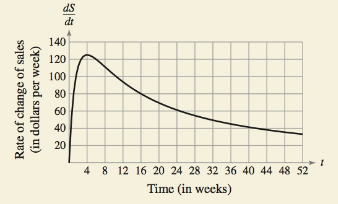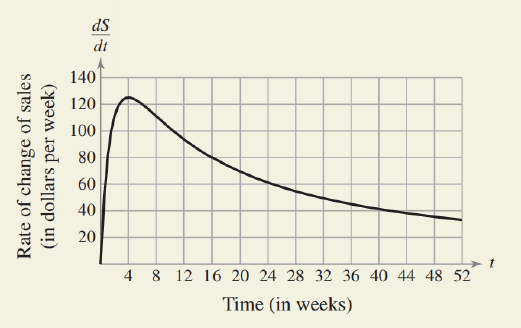Chapter 6.2, Problem 60E### Calculus: An Applied Approach (Min...

10th Edition
Ron Larson
ISBN: 9781305860919

#### Solutions

Chapter
Section### Calculus: An Applied Approach (Min...

10th Edition
Ron Larson
ISBN: 9781305860919
Textbook Problem
1 views

# HOW DO YOU SEE IT? The graph shows the rate of change of the sales of a new product.(a) Approximate the rate of change of the sales after 16 weeks. Explain your reasoning.(b) Approximate the weeks for which the sales are increasing. Explain your reasoning.

(a)

To determine

The rate of change of sales after 16 weeks, with the help of the provided graph of rate of change of the sales for a new product, which is shown below as;Explanation

Given Information:

The provided graph of rate of change of the sales for a new product, which is shown below as:

The provided graph of rate of change of the sales for a new product, which is shown below as:

From above graph, it can be clearly seen that for t=16<

(b)

To determine

Which weeks, Sales are increasing with the help of the provided graph of rate of change of the sales for a new product, which is shown below as:### Still sussing out bartleby?

Check out a sample textbook solution.

See a sample solution

#### The Solution to Your Study Problems

Bartleby provides explanations to thousands of textbook problems written by our experts, many with advanced degrees!

Get Started

#### In Exercises 516, evaluate the given quantity. log1/212

Finite Mathematics and Applied Calculus (MindTap Course List)

#### Intervals Graph the set. 65. (, 4) (4, )

Precalculus: Mathematics for Calculus (Standalone Book)

#### 23. If and , find (a) (b) (c) (d)

Mathematical Applications for the Management, Life, and Social Sciences

#### True or False: If h'(x) = k(x), then k(x) is an antiderivative of h(x).

Study Guide for Stewart's Single Variable Calculus: Early Transcendentals, 8th

#### Distinguish between a hypothesis and a prediction.

Research Methods for the Behavioral Sciences (MindTap Course List)